# Diagonalizing a matrix NOT having full rank, what does it mean?

This is going to be a quick intuition about what it means to diagonalize a matrix that does not have full rank (i.e. has null determinant).

Every matrix can be seen as a linear map between vector spaces. Stating that a matrix is similar to a diagonal matrix equals to stating that there exists a basis of the source vector space in which the linear transformation can be seen as a simple stretching of the space, as re-scaling the space. In other words, diagonalizing a matrix is the same as finding an orthogonal grid that is transformed in another orthogonal grid. I recommend this article from AMS for good visual representations of the topic.

### Diagonalization on non full rank matrices

That’s all right – when we have a matrix from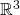in, if it can be diagonalized, we can find a basis in which the transformation is a re-scaling of the space, fine.

But what does it mean to diagonalize a matrix that has null determinant? The associated transformations have the effect of killing at least one dimension: indeed, axmatrix of rank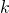has the effect of lowering the output dimension by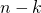. For example, a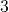xmatrix of rank 2 will have an image of size 2, instead of 3. This happens because two basis vectors are merged in the same vector in the output, so one dimension is bound to collapse.

Let’s consider the sample matrix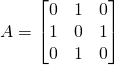which has non full rank because has two equal rows. Indeed, one can check that the two vectorsgo in the same basis vector. This means thatinstead of 3. In fact, it is common intuition that when the rank is not full, some dimensions are lost in the transformation. Even if it’s axmatrix, the output only has 2 dimensions. It’s like at the end of Inception when the 4D space in which cooper is floating gets shut.

However,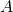is also a symmetric matrix, so from the spectral theorem we know that it can be diagonalized. And now to the vital questions: what do we expect? What meaning does it have? Do we expect a basis of three vectors even if the map destroys one dimension?

Pause and ponder.

Diagonalize the matrixand, indeed, you obtain three eigenvalues: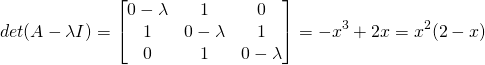The eigenvalues are thus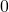,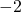and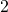, each giving a different eigenvector.  Taken all together, they form a orthogonal basis of. The fact thatis among the eigenvalues is important: it means that all the vectors belonging to the associated eigenspace all go to the same value: zero. This is the mathematical representation of the fact that one dimension collapses.

At first, I naively thought that, since the transformation destroys one dimension, I should expect to find a 2D basis of eigenvectors. But this was because I confused the source of the map with its image! The point is that we can still find a basis of the source space from the perspective of which the transformation is just a re-scaling of the space. However, that doesn’t tell anything about the behavior of the transformation, whether it will preserve all dimensions: it is possible that two vectors of the basis will go to the same vector in the image!

In fact, the fact that the matrixhas the first and third rows that are the same means that the basis vectors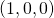and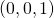both go into. A basis of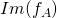is simply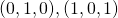, and we should not be surprised by the fact that those vectors have three entries. In fact, two vectors (even with three coordinates) only allow to represent a 2D space. In theory, one could express any vector that is combination of the basis above as combination of the usual 2D basis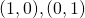, to confirm that.